# Introduction

For many species, count data is very skewly distributed. This is especially the case for species which tend to flock or cluster together at one or more resting places, that may or may not change from year to year. Especially when these places do change, it will become difficult for rtrim (or any GLM) to model this correctly, because the episodic high counts are not captured well in a site factor, nor in a time-point factor. The result is that model deviations for these place/time combinations are large, which for serveral resons may affect the computed overdispersion. To some extent, this effect will be true (because of the larger-than-expected variance), but there is also the risk of methodologival artefacts, which we would like to avoid.

This vigenette aims as understanding the nature of huge overdispersion for these cases, and presents a number of mitigating approaches implemented in rtrim.

Let’s look at an example for Oystercatcher data that comes with RTRIM. First we plot the sorted raw counts.

library(rtrim)
data(oystercatcher)

# Collect all raw count data
ok <- is.finite(oystercatcher$count) & oystercatcher$count > 0
count <- oystercatcher$count[ok] plot(count, type='p', pch=16, col="red", cex=0.4)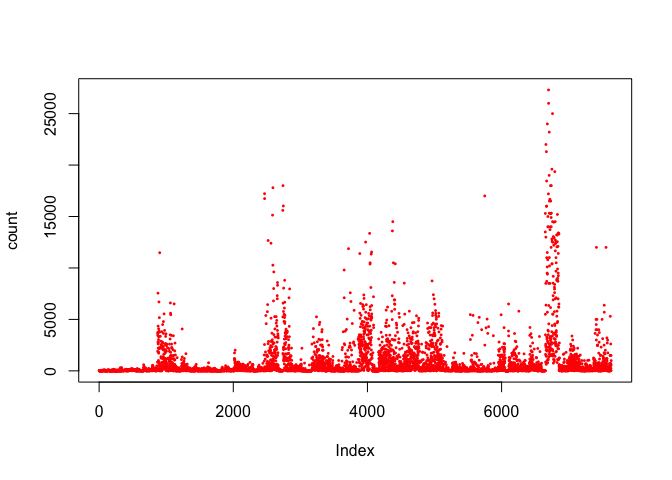Of course, sorting does help to see the big picture. count <- sort(count) plot(count/1000, type='p', pch=16, col="red", cex=0.4, las=TRUE, ylab="count (x1000)")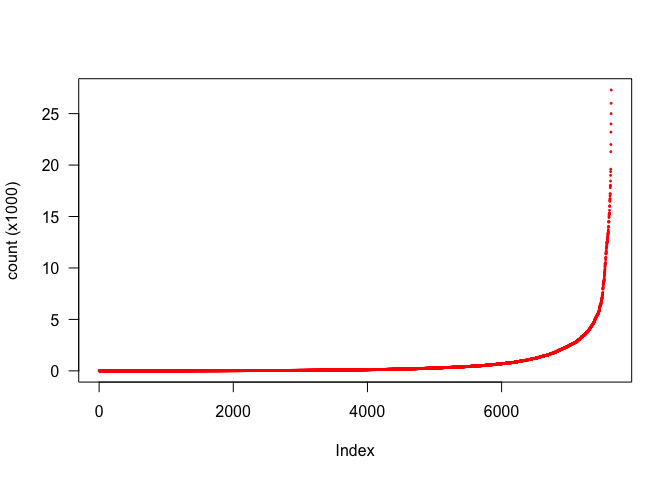So it appears that a few site/year/month combinations have the majority of all individuals. We can plot exactly this: cum_count <- cumsum(sort(count, decreasing = TRUE)) # cumulative counts, largest first cum_pct <- 100 * cum_count / sum(count) # express as percentage of total n <- length(count) obs_pct <- 100 * (1:n)/n plot(obs_pct, cum_pct, type='n', xlab="Observations (%)", ylab="Cum. counts (%)", las=1) points(obs_pct, cum_pct, pch=16, cex=0.3, col="red") abline(a=100, b=-1, lty="dashed") grid()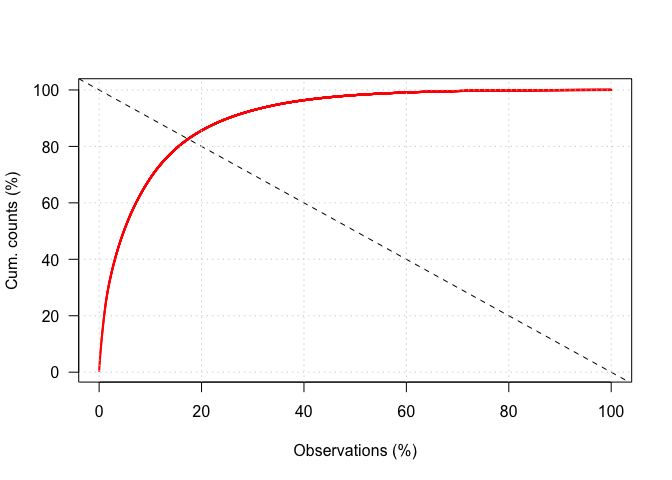In this case, we see the typical “Pareto-principle”: 20% of the data points represent 80% of the total counts. This is likely to have a strong impact on estimated overdispersion. # Overdispersion for clustered observations: the problem Let’s see how large overdispersion actually is. Because using the full dataset may be a bit slow for use within a vignette, we create a second dataset using only the last 10 year, and the sites that have the best coverage (percentage of years and months that have positive counts). oystercatcher2 <- subset(oystercatcher, year>=2005) calc_coverage <- function(x) 100*mean(is.finite(x) & x>0) coverage <- aggregate(count ~ site, data=oystercatcher2, calc_coverage, na.action=na.pass) coverage <- coverage[order(coverage$count, decreasing=TRUE), ]
plot(coverage$count, ylab="coverage (%)", pch=16, col=gray(0,0.5), las=1) abline(a=50, b=0, col="red")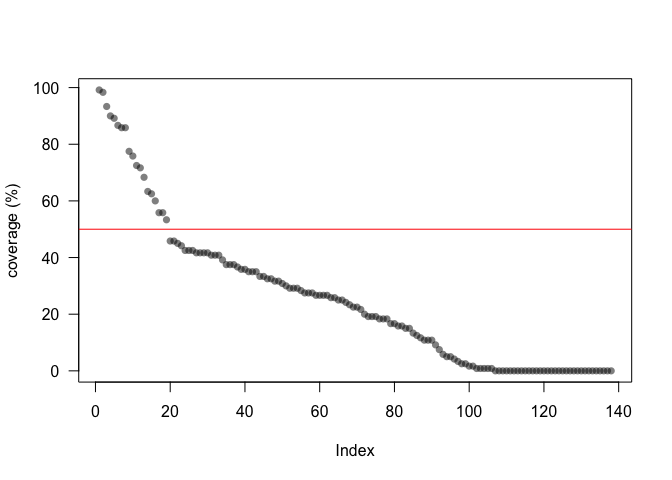Based on above figure, we decide to use a threshold of 50% coverage, which is about 20 sites. ok <- subset(coverage, count > 45) oystercatcher3 <- subset(oystercatcher2, site %in% ok$site)
z <- trim(count ~ site + (year+month), data=oystercatcher3, model=3, overdisp=TRUE)
summary(z)
#> Call:
#> tools::buildVignettes(dir = ".", tangle = TRUE)
#>
#> Model  : 3
#> Method : GEE (Convergence reached after 8 iterations)
#>
#> Coefficients:
#> 1   year     1  0.00000000 0.00000000 1.0000000 0.00000000
#> 2   year     2 -0.12910821 0.10412101 0.8788789 0.09150975
#> 3   year     3 -0.26286715 0.10480722 0.7688440 0.08058041
#> 4   year     4 -0.34053864 0.10443466 0.7113870 0.07429346
#> 5   year     5 -0.40775682 0.11236331 0.6651406 0.07473740
#> 6   year     6 -0.23146611 0.10398967 0.7933696 0.08250224
#> 7   year     7 -0.45102143 0.10950416 0.6369772 0.06975165
#> 8   year     8 -0.05673496 0.09844313 0.9448445 0.09301345
#> 9   year     9 -0.31097791 0.10313944 0.7327301 0.07557337
#> 10  year    10 -0.23289935 0.10190991 0.7922333 0.08073643
#> 11 month     1  0.00000000 0.00000000 1.0000000 0.00000000
#> 12 month     2  0.05336631 0.09636071 1.0548160 0.10164281
#> 13 month     3 -0.62159914 0.11900349 0.5370849 0.06391497
#> 14 month     4 -1.15666563 0.14573389 0.3145332 0.04583815
#> 15 month     5 -1.59739827 0.16395820 0.2024225 0.03318883
#> 16 month     6 -1.45125447 0.16571737 0.2342762 0.03882364
#> 17 month     7 -0.66394637 0.12149335 0.5148157 0.06254668
#> 18 month     8  0.03478200 0.10172791 1.0353940 0.10532846
#> 19 month     9  0.09859027 0.09225028 1.1036140 0.10180870
#> 20 month    10 -0.12836654 0.10233123 0.8795309 0.09000348
#> 21 month    11  0.07262761 0.09633538 1.0753300 0.10359232
#> 22 month    12  0.03298819 0.10082010 1.0335383 0.10420144
#>
#>  Overdispersion     : 1213.0781
#>
#> Goodness of fit:
#>               Chi-square = 2549890.26, df=2102, p=0.0000
#>         Likelihood Ratio = 1613397.34, df=2102, p=0.0000
#>   AIC (up to a constant) = 1609193.34

So overdispersion is indeed huge!

You may recall from Models and statistical methods in rtrim that the formula for overdispersion is given by $\sigma^2 = \frac{1}{n-p} \sum_{ijm} r^2_{ijm}$ with $$n$$ the number of observations, $$p$$ the number of model parameters and $$r$$ Pearson residuals, given by $r = \frac{f_{ijm} -\mu_{ijm}}{\sqrt{\mu_{ijm}}}.$

Species like Oystercatcher are known to cluster in winter: many individuals may appear at one monitoring site at one time, and at another site at another time. This clustering behaviour is unlikely to be captured in full by the TRIM model. Therefore, residuals (i.e. $$f-\mu$$) may be large, and more than proportionally affect overdispersion $$\sigma^2$$ because the nonlinearity involved (i.e. the squaring process). So, while overdispersion may be high, it is also very likely to be overestimated.

We can see the effect of large deviations on the computed overdispersion by skipping the 0,1,2,etc largest values from the computation.

# Retrieve raw observed and modelled counts
out <- results(z)
ok  <- is.finite(out$observed) f <- out$observed[ok]
mu  <- out$fitted[ok] # Compute Pearson residuals, and sort r <- (f - mu) / sqrt(mu) idx <- order(r^2, decreasing=TRUE) r <- r[idx] # How many obervations and parameters? n <- length(f) p <- z$nsite + z$nyear-1 + z$nmonth-1

# Set up
skips <- 0 : (n %/% 2) # skip none to approx 50% of all residuals
sig2 <- numeric(length(skips)) # Allocate a vector with the computed overdispersion
for (i in seq_along(skips)) {
r2 <- r[skips[i] : n]^2
df <- n - p - skips[i] # correct for skipped
sig2[i] <- sum(r2) / df
}
plot(skips, sig2, type='l', col="red", las=1)
abline(h=0.0, lty="dashed", col="red")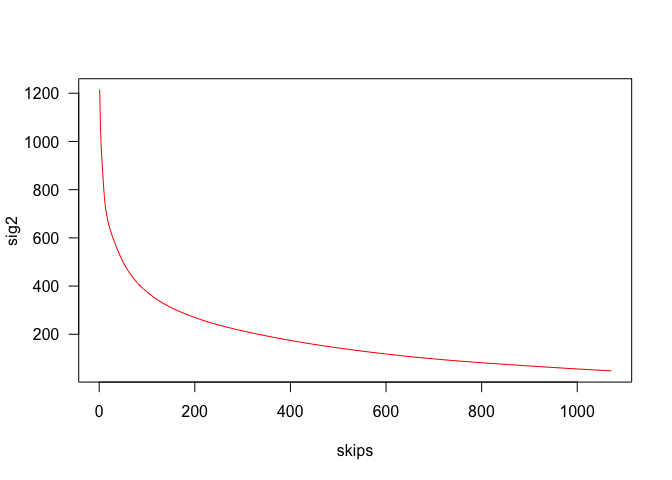Indeed, overdispersion appears to be very sensitive for the largest residuals, suggesting that the actual value is not very robust against the contingent observations.

The question now is, how to compute a more robust and realistic estimate of overdispersion, which may be (much) larger than 1, but not affected by artefacts resulting from the estimation procedure?

# Approach #1: average first, square later.

The formal approach to compute overdispersion is to first square the residuals, and then do the averaging, which is very sensitive for outliers. Let’s see what happens if we reverse this order: first we do the averaging (using the absolute values of the residuals), then the squaring. Again, formally this is not correct, but at least if gives us some hint whether this phenomenon is the cause of the problem.

sig2_alt1 <- numeric(length(skips))
for (i in seq_along(skips)) {
rr <- r[skips[i] : n]
df <- n - p - skips[i] # correct for skipped
sig2_alt1[i] <- (sum(abs(rr))/df)^2
}
plot(skips, sig2, type='l', col="red", las=1)
lines(skips, sig2_alt1, col="blue")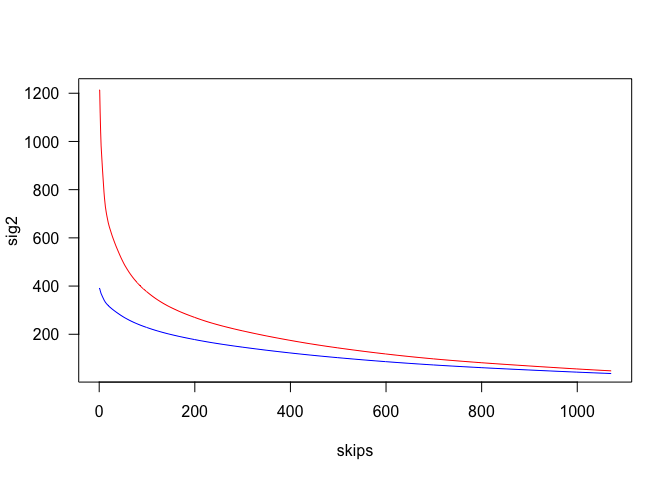Indeed, it appears that the effect is strongly mitigated reducing $$\sigma^2$$ from about 850 to about 200, and converges to about the same value once all outliers have beem removed. But again, this approach is formally incorrect.

# Approach #2: remove outliers (nonparametric)

A second approach is to just remove the outliers. One way to do this is to use a nonparametric methods, such as based on the interquantile interval (Tuckey’s Fence’)

# residuals, and their square
r <- (f - mu) / sqrt(mu)
r2 <- r^2

# classic overdispersion
n <- length(f)
p <- z$nsite + z$nyear-1 + z\$nmonth-1
sig2_std <- sum(r^2) / (n-p)

# Compute interquantile distance and outlier limits
Q <- quantile(r2, c(0.25, 0.50, 0.75)) # such that Q is what you expect
IQR <- (Q-Q) # Interquartile range
k <- 3 # Tuckey's criterion for "far out"
lo <- Q - k * IQR # low threshold value added for completeless only
hi <- Q + k * IQR
cat(sprintf("Using r2 limit %f -- %f\n", lo, hi))
#> Using r2 limit -1463.172751 -- 2039.455264
nlo <- sum(r2<lo)
cat(sprintf("  removing %d lower outliers (%.1f%%)\n", nlo, 100*nlo/length(f)))
#>   removing 0 lower outliers (0.0%)
nhi <- sum(r2>hi)
cat(sprintf("  removing %d upper outliers (%.1f%%)\n", nhi, 100*nhi/length(f)))
#>   removing 165 upper outliers (7.7%)
ok <- (r2>lo) & (r2<hi)
df <- length(r2) - p
sig2_alt2 <- sum(r2[ok]) / df
cat(sprintf("Reduced sig2 from %.1f to %.1f\n", sig2_std, sig2_alt2))
#> Reduced sig2 from 1213.1 to 272.9

So, this approach appears to work. It is implemented in R-TRIM using the constrain_overdisp argument, where values ($$>1$$) represents the IQR multiplier (defaulting to 3, for far out’, in above example)

z <- trim(..., overdisp=TRUE, constrain_overdisp=3, ...)

# Approach #3: remove outliers (parametric)

One of the assumptions behind TRIM is that the residuals are approximately $$\chi^2$$-distributed. Thus, it makes sense to fit such a distribution to find the, say, 99% percentile, using an estimate of $$\sigma^2$$ as a scaling parameter, to obtain a threshold value to identify outliers. Since the value of $$\sigma^2$$ will depend on the outliers removed, an iterative approach is required.

level <- 0.99
niter <- 10
sig2_alt3 <- numeric(niter)
ok <- !logical(length(r2)) # all TRUE
for (i in 1:niter) {
# Compute current overdispersion
df <- sum(ok) - p
sig2_alt3[i] <- sum(r2[ok]) / df
# Compute new cutoff value
cutoff <- sig2_alt3[i] * qchisq(level, 1)
ok <- r2 < cutoff
}
ntotal <- length(r2)
noutlier <- ntotal - sum(ok)
cat(sprintf("Removed %d outliers (%.1f%%)\n", noutlier, 100*noutlier/ntotal))
#> Removed 166 outliers (7.7%)
plot(sig2_alt3, type='l', col="red", ylim=range(0,range(sig2_alt3)), las=1)
points(sig2_alt3, pch=16, col="red")
text(25,400, sprintf("Convergence at %.1f", sig2_alt3[niter]))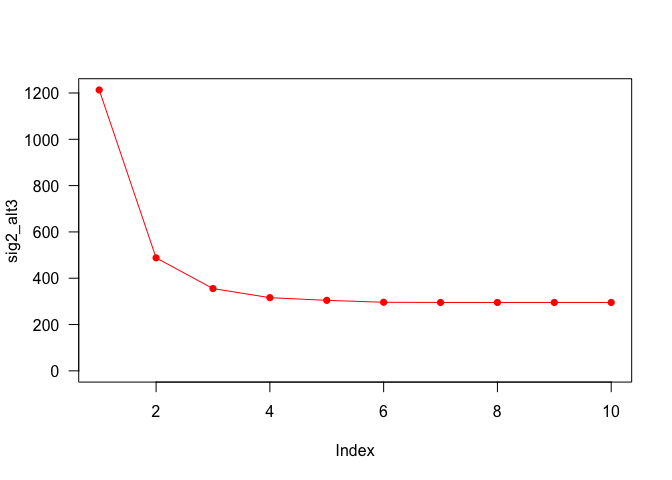Again, this method works well, and is implemented in R-TRIM using the constrain_overdisp option, for values in the range $$0\ldots1$$, e.g.

z <- trim(..., overdisp=TRUE, constrain_overdisp=0.99, ...)

Note that constrain_overdisp has 3 possible values:

• $$0 \ldots 1$$ : Using Chi-squared outlier detection, with the specified level.
• $$1$$ : No constraints.
• $$>1$$ : Using Tuckey’s Fence, with the specified IQR multiplier.

# Application

Here is an example where we compare unconstrained / constrained overdispersion, using the 3th approach.

z1 <- trim(count ~ site + (year+month), data=oystercatcher3, model=3,
overdisp=TRUE, constrain_overdisp=0.99)
z2 <- trim(count ~ site + (year+month), data=oystercatcher3, model=3,
overdisp=TRUE, constrain_overdisp=1)
idx1 <- index(z1)
idx2 <- index(z2)
plot(idx1, idx2, names=c("constrained","unconstrained"))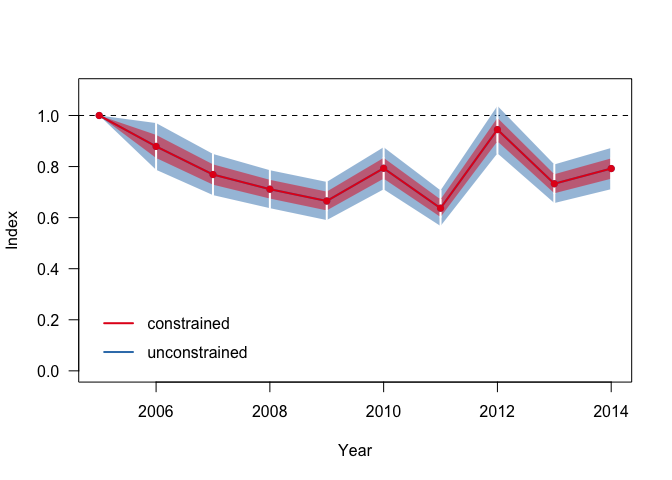Where it is clear that the standard errors for the constrained overdispersion run are considerable smaller.